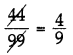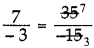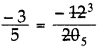# DAV Class 7 Maths Chapter 1 Worksheet 2 Solutions

The DAV Class 7 Maths Book Solutions Pdf and DAV Class 7 Maths Chapter 1 Worksheet 2 Solutions of Rational Numbers offer comprehensive answers to textbook questions.

## DAV Class 7 Maths Ch 1 WS 2 Solutions

Question 1.
In each of the following cases, show that the rational numbers are equivalent.
(i) $$\frac{4}{9}$$ and $$\frac{44}{99}$$
$$\frac{4}{9}$$ and $$\frac{44}{99}$$$$\frac{4}{9}$$ = $$\frac{4}{9}$$ hence they are equivalent rational numbers.

(ii) $$\frac{7}{-3}$$ and $$\frac{35}{-15}$$
$$\frac{7}{-3}$$ and $$\frac{35}{-15}$$$$\frac{7}{-3}$$ = $$\frac{7}{-3}$$, So, they are equivalent rational numbers.

(iii) $$\frac{-3}{5}$$ and $$\frac{-12}{20}$$
$$\frac{-3}{5}$$ and $$\frac{-12}{20}$$$$\frac{-3}{5}$$ = $$\frac{-3}{5}$$ So, they are not equivalent rational numbers.

Question 2.
In each of the following cases, show that the rational numbers are not equivalent.
(i) $$\frac{4}{9}$$ and $$\frac{16}{27}$$
$$\frac{4}{9}$$ and $$\frac{16}{27}$$
⇒ 4 × 27 and 16 × 9
⇒ 108 ≠ 144

(ii) $$\frac{-100}{3}$$ and $$\frac{300}{9}$$
$$\frac{-100}{3}$$ and $$\frac{300}{9}$$
⇒ -100 × 9 and 300 × 3
⇒ -900 ≠ 900

(iii) $$\frac{3}{-17}$$ and $$\frac{8}{-51}$$
$$\frac{3}{-17}$$ and $$\frac{8}{-51}$$
⇒ 3 × (-51) and 8 × (-17)
⇒ -153 ≠ -136
So, they are not equivalent rational numbers.Question 3.
Write three rational numbers equivalent to each of the following:
(i) $$\frac{4}{7}$$
$$\frac{4}{7}=\frac{4 \times 2}{7 \times 2}=\frac{8}{14}$$
and $$\frac{4}{7}=\frac{4 \times 3}{7 \times 3}=\frac{12}{21}$$ and $$\frac{4 \times 4}{7 \times 4}=\frac{16}{28}$$

Hence, the three equivalent fractions are
$$\frac{8}{14}=\frac{12}{21}=\frac{16}{28}$$

(ii) $$\frac{36}{108}$$
$$\frac{36}{108}=\frac{36 \div 2}{108 \div 2}=\frac{18}{54}$$
and $$\frac{36 \div 3}{108 \div 3}=\frac{12}{36}$$ and $$\frac{36 \div 4}{108 \div 4}=\frac{9}{27}$$

Hence, the equivalent fractions are
$$\frac{18}{54}, \frac{12}{36}$$ and 

(iii) $$\frac{-5}{-7}$$
$$\frac{-5}{-7}=\frac{-5 \times 2}{-7 \times 2}=\frac{-10}{-14}$$
and $$\frac{-5 x-2}{-7 x-2}=\frac{10}{14}$$ and $$-\frac{5 \times 3}{-7 \times 3}=\frac{-15}{-21}$$

Hence, the equivalent fractions are
$$\frac{-10}{-14}, \frac{10}{14}$$ and $$\frac{-15}{-21}$$

(iv) $$\frac{-72}{180}$$
$$\frac{-72}{180}=\frac{-72 \div 2}{180 \div 2}=\frac{-36}{90}$$
and $$\frac{-72 \div 3}{180 \div 3}=\frac{-24}{60}$$ and $$\frac{-72 \div 4}{180 \div 4}=\frac{-18}{45}$$

Hence the equivalent rational numbers are
$$\frac{-36}{90}, \frac{-24}{60}$$ and $$\frac{-18}{45}$$

Question 4.
Express $$\frac{3}{5}$$ as rational number with numerator,
(i) -21
$$\frac{3}{5}=\frac{3 \times-7}{5 \times-7}$$
= $$\frac{-21}{-35}$$

(ii) 150
$$\frac{3}{5}=\frac{3 \times 50}{5 \times 50}$$
= $$\frac{150}{250}$$

Question 5.
Express $$\frac{4}{-7}$$ as a rational number with denominator
(i) 84
$$\frac{4}{-7}=\frac{4 \times-12}{-7 x-12}$$
= $$\frac{-48}{84}$$

(ii) -28
$$\frac{4}{-7}=\frac{4 \times 4}{-7 \times 4}$$
= $$\frac{16}{-28}$$

Question 6.
Express $$\frac{90}{216}$$ as a rational number with numerator 5.
$$\frac{90}{216}=\frac{90 \div 18}{216 \div 18}$$
= $$\frac{5}{12}$$

Question 7.
Express $$\frac{-64}{256}$$ as a rational number with denominator 8.
$$\frac{-64}{256}=\frac{-64 \div 32}{256 \div 32}$$
= $$\frac{-2}{8}$$Question 8.
Find the equivalent forms of the rational numbers having a common denominator in each of the following collections of rational numbers:
(i) $$\frac{2}{5}, \frac{6}{13}$$
The L.C.M of 5 and 13 = 5 × 13 = 65
= $$\frac{2 \times 13}{5 \times 13}, \frac{6 \times 5}{13 \times 5}$$
⇒ $$\frac{26}{65}, \frac{30}{65}$$

(ii) $$\frac{1}{7}, \frac{2}{8}, \frac{3}{14}$$
= $$\frac{1}{7} \times \frac{8}{8}, \frac{2 \times 7}{8 \times 7}, \frac{3 \times 4}{14 \times 4}$$
⇒ $$\frac{8}{56}, \frac{14}{56}, \frac{12}{56}$$
(iii) $$\frac{5}{12}, \frac{7}{4}, \frac{9}{60}, \frac{11}{3}$$
= $$\frac{5}{12} \times \frac{5}{5}, \frac{7}{4} \times \frac{15}{15}, \frac{9}{60} \times \frac{1}{1}, \frac{11}{3} \times \frac{20}{20}$$
= $$\frac{25}{60}, \frac{105}{60}, \frac{9}{60}, \frac{220}{60}$$